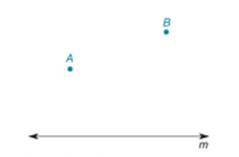Chapter 3.3, Problem 17E### Elementary Geometry for College St...

6th Edition
Daniel C. Alexander + 1 other
ISBN: 9781285195698

#### Solutions

Chapter
Section### Elementary Geometry for College St...

6th Edition
Daniel C. Alexander + 1 other
ISBN: 9781285195698
Textbook Problem
1 views

# In Exercises 13 to 18, describe the line segments as determined, undetermined, or overdetermined. Use the accompanying drawing for reference.Draw a line from A perpendicular to m .

To determine

To draw:

The line from A perpendicular to line m and describe it as determined, underdetermined, or over-determined.

Explanation

Given:

The given figure is as follows.

Figure (1)

Approach:

If there is exactly one possibility for occurrence of a line segment, it is considered as determined segment

Calculation:

Consider the given figure.

### Still sussing out bartleby?

Check out a sample textbook solution.

See a sample solution

#### The Solution to Your Study Problems

Bartleby provides explanations to thousands of textbook problems written by our experts, many with advanced degrees!

Get Started

#### Find more solutions based on key concepts